## 2.4.1 A STRIPS-Like Representation

STRIPS-like representations have been the most common logic-based representations for discrete planning problems. This refers to the STRIPS system, which is considered one of the first planning algorithms and representations ; its name is derived from the STanford Research Institute Problem Solver. The original representation used first-order logic, which had great expressive power but many technical difficulties. Therefore, the representation was later restricted to only propositional logic , which is similar to the form introduced in this section. There are many variations of STRIPS-like representations. Here is one formulation:

Formulation 2..4 (STRIPS-Like Planning)
1. A finite, nonempty set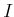of instances.
2. A finite, nonempty setof predicates, which are binary-valued (partial) functions of one of more instances. Each application of a predicate to a specific set of instances is called a positive literal. A logically negated positive literal is called a negative literal.
3. A finite, nonempty set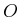of operators, each of which has: 1) preconditions, which are positive or negative literals that must hold for the operator to apply, and 2) effects, which are positive or negative literals that are the result of applying the operator.
4. An initial set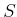which is expressed as a set of positive literals. Negative literals are implied. For any positive literal that does not appear in, its corresponding negative literal is assumed to hold initially.
5. A goal set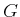which is expressed as a set of both positive and negative literals.

Formulation 2.4.1 provides a definition of discrete feasible planning expressed in a STRIPS-like representation. The three most important components are the sets of instances, predicates, and operators. Informally, the instances characterize the complete set of distinct things that exist in the world. They could, for example, be books, cars, trees, and so on. The predicates correspond to basic properties or statements that can be formed regarding the instances. For example, a predicate called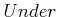might be used to indicate things like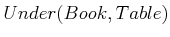(the book is under the table) or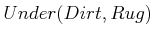. A predicate can be interpreted as a kind of function that yields TRUE or FALSE values; however, it is important to note that it is only a partial function because it might not be desirable to allow any instance to be inserted as an argument to the predicate.

If a predicate is evaluated on an instance, for example,, the expression is called a positive literal. The set of all possible positive literals can be formed by applying all possible instances to the domains over which the predicates are defined. Every positive literal has a corresponding negative literal, which is formed by negating the positive literal. For example,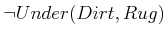is the negative literal that corresponds to the positive literal, and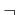denotes negation. Let a complementary pair refer to a positive literal together with its counterpart negative literal. The various components of the planning problem are expressed in terms of positive and negative literals.

The role of an operator is to change the world. To be applicable, a set of preconditions must all be satisfied. Each element of this set is a positive or negative literal that must hold TRUE for the operator to be applicable. Any complementary pairs that can be formed from the predicates, but are not mentioned in the preconditions, may assume any value without affecting the applicability of the operator. If the operator is applied, then the world is updated in a manner precisely specified by the set of effects, which indicates positive and negative literals that result from the application of the operator. It is assumed that the truth values of all unmentioned complementary pairs are not affected.

Multiple operators are often defined in a single statement by using variables. For example,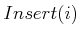may allow any instance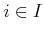to be inserted. In some cases, this dramatically reduces the space required to express the problem.

The planning problem is expressed in terms of an initial setof positive literals and a goal setof positive and negative literals. A state can be defined by selecting either the positive or negative literal for every possible complementary pair. The initial setspecifies such a state by giving the positive literals only. For all possible positive literals that do not appear in, it is assumed that their negative counterparts hold in the initial state. The goal setactually refers to a set of states because, for any unmentioned complementary pair, the positive or negative literal may be chosen, and the goal is still achieved. The task is to find a sequence of operators that when applied in succession will transform the world from the initial state into one in which all literals ofare TRUE. For each operator, the preconditions must also be satisfied before it can be applied. The following example illustrates Formulation 2.4.

Example 2..6 (Putting Batteries into a Flashlight)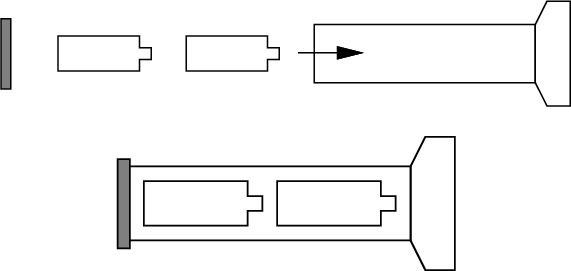Imagine a planning problem that involves putting two batteries into a flashlight, as shown in Figure 2.17. The set of instances are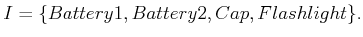(2.21)

Two different predicates will be defined,and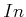, each of which is a partial function on. The predicatemay only be applied to evaluate whether the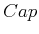isthe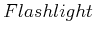and is written as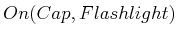. The predicatemay be applied in the following two ways: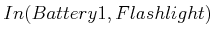,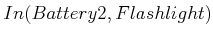, to indicate whether either battery is in the flashlight. Recall that predicates are only partial functions in general. For the predicate, it is not desirable to apply any instance to any argument. For example, it is meaningless to define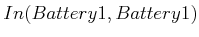and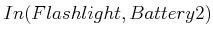(they could be included in the model, always retaining a negative value, but it is inefficient).

The initial set is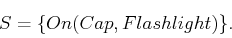(2.22)

Based on, both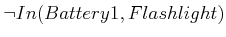and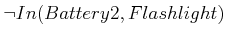are assumed to hold. Thus,indicates that the cap is on the flashlight, but the batteries are outside.

The goal state is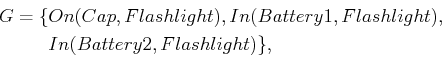(2.23)

which means that both batteries must be in the flashlight, and the cap must be on.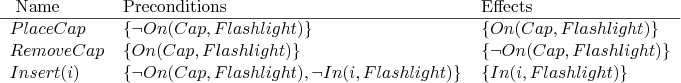The setconsists of the four operators, which are shown in Figure 2.18. Here is a plan that reaches the goal state in the smallest number of steps: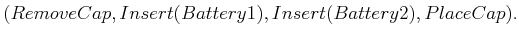(2.24)

In words, the plan simply says to take the cap off, put the batteries in, and place the cap back on.

This example appears quite simple, and one would expect a planning algorithm to easily find such a solution. It can be made more challenging by adding many more instances to, such as more batteries, more flashlights, and a bunch of objects that are irrelevant to achieving the goal. Also, many other predicates and operators can be added so that the different combinations of operators become overwhelming.A large number of complexity results exist for planning expressed using logic. The graph search problem is solved efficiently in polynomial time; however, a state transition graph is not given as the input. An input that is expressed using Formulation 2.4 may describe an enormous state transition graph using very few instances, predicates, and operators. In a sense, the model is highly compressed when using some logic-based formulations. This brings it closer to the Kolmogorov complexity [248,630] of the state transition graph, which is the shortest bit size to which it can possibly be compressed and then fully recovered by a Turing machine. This has the effect of making the planning problem appear more difficult. Concise inputs may encode very challenging planning problems. Most of the known hardness results are surveyed in Chapter 3 of . Under most formulations, logic-based planning is NP-hard. The particular level of hardness (NP, PSPACE, EXPTIME, etc.) depends on the precise problem conditions. For example, the complexity depends on whether the operators are fixed in advance or included in the input. The latter case is much harder. Separate complexities are also obtained based on whether negative literals are allowed in the operator effects and also whether they are allowed in preconditions. The problem is generally harder if both positive and negative literals are allowed in these cases.

Steven M LaValle 2020-08-14